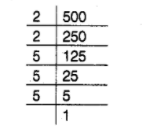# Show that 500 is not a perfect square.

Question:

Show that 500 is not a perfect square.

Solution:

Resolving 500 into prime factors, we have$500=2 \times 2 \times 5 \times 5 \times 5$

Grouping the factors into pairs of equal factors, we get

$500=(2 \times 2) \times(5 \times 5 \times 5)$

Clearly, by grouping into pairs of equal factors, we are left with one factor 5 , which cannot be paired.

Hence, 500 is not a perfect square.